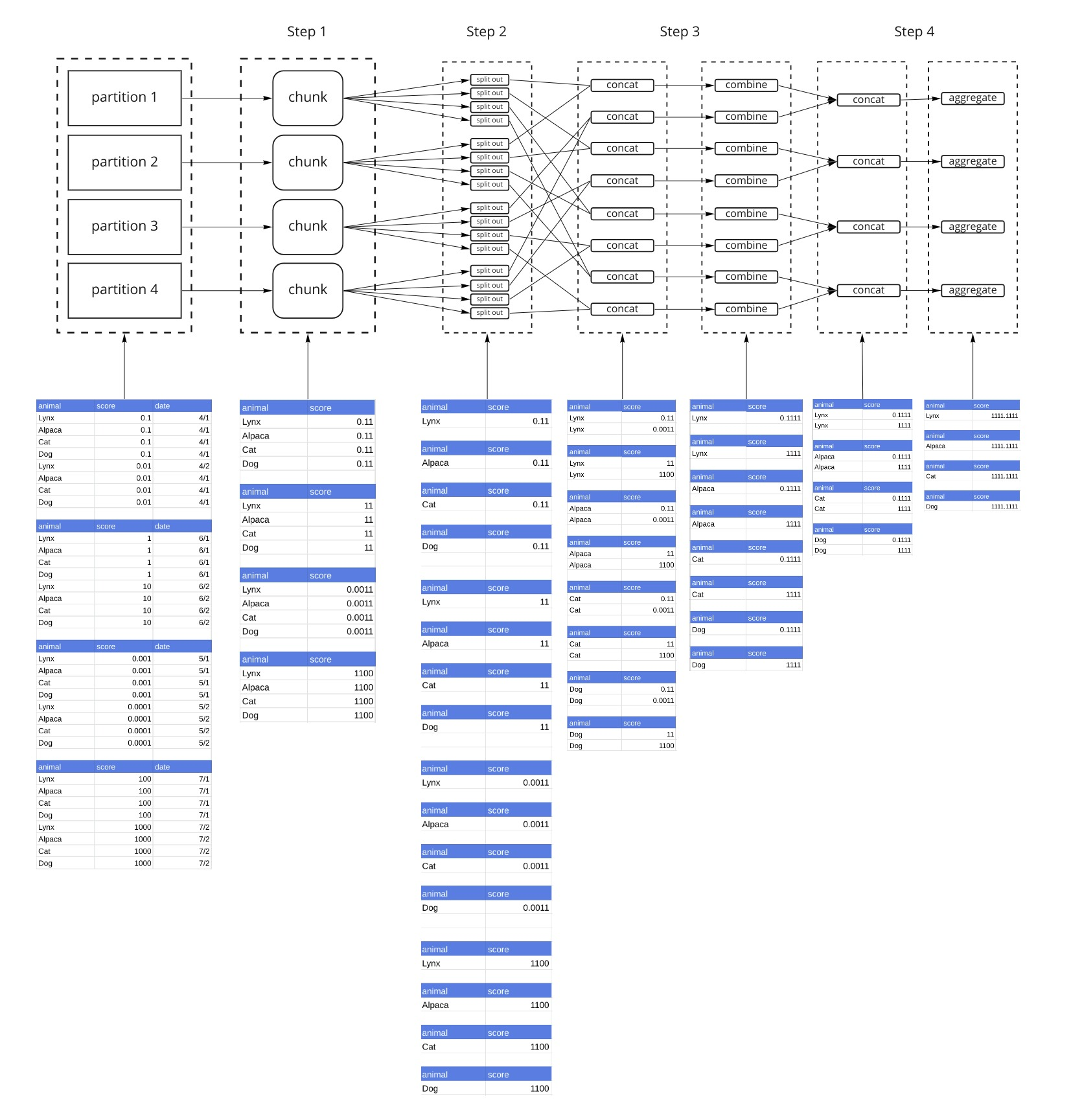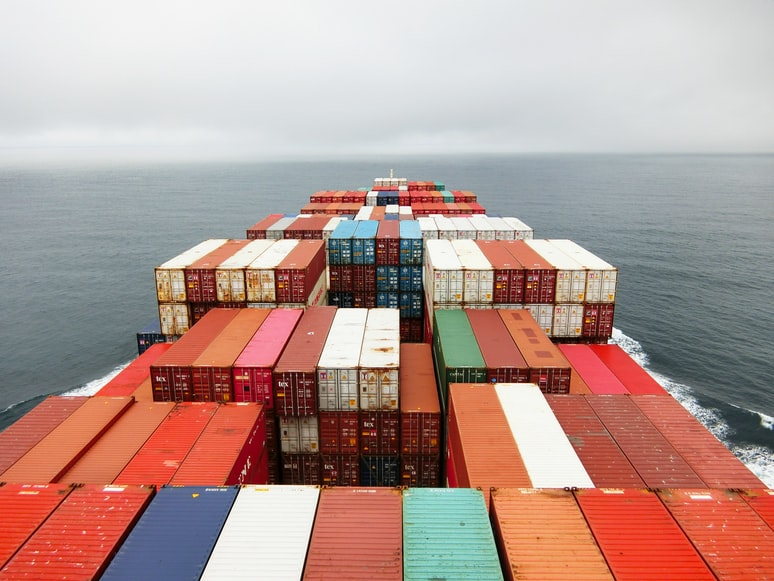# Dask DataFrame is not Pandas

This article is the second article of an ongoing series on using Dask in practice. Each article in this series will be simple enough for beginners, but provide useful tips for real work. The next article in the series is about parallelizing for loops, and other embarrassingly parallel operations with dask.delayed.

By Hugo Shi, Founder of Saturn Cloud

## The Allure

You start with medium-sized data sets. Pandas does quite well. Then the data sets get larger, and so you scale up to a larger machine. But eventually, you run out of memory on that machine, or you need to find a way to leverage more cores because your code is running slowly. At that point, you replace your Pandas DataFrame object with a Dask DataFrame.

Unfortunately, this doesn’t usually go well, and results in a good amount of pain. Either some of the methods you rely on in Pandas, are not implemented in Dask DataFrame (I’m looking at you, MultiIndex), the behavior of the methods is slightly different, or the corresponding Dask operation fails, runs out of memory and crashes (I thought it wasn’t supposed to do that!)

Pandas is a library designed for a single Python process. Distributed algorithms and data structures are fundamentally different. There is work that can be done on the Dask DataFrame side to make this better, but single processes, and clusters of machines, will always have very different performance characteristics. You should not try to fight this fundamental truth.

Dask is a great way to scale up your Pandas code. Naively converting your Pandas DataFrame into a Dask DataFrame is not the right way to do it. The fundamental shift should not be to replace Pandas with Dask, but to re-use the algorithms, code, and methods you wrote for a single Python process. That’s the meat of this article. Once you reframe your thinking, the rest isn’t rocket science.

There are 3 main ways to leverage your Pandas code with Dask

• Break up your big problem into many small problems
• Use group by and aggregations
• Use dask dataframes as a container for other distributed algorithms.

## Break up your big problem into many small problemsA Dask DataFrame is made up of many Pandas DataFrames. It’s really good at moving rows from those Pandas DataFrames around, so that you can use them in your own functions. The general approach here is to express your problem in a split-apply-combine pattern.

• Split your big dataset (Dask DataFrame) into smaller datasets (Pandas DataFrame)
• Apply a function (a Pandas function, not a Dask function) to those smaller datasets
• Combine the results back into a big dataset (Dask DataFrame)

There are 2 main approaches to splitting your data:

`set_index` will make one column of the Dask DataFrame the index, and sort the data according to that index. It will by default estimate the data distribution of that column so that you end up with evenly sized partitions (Pandas DataFrames).

`shuffle` will group rows together, so that rows with the same values for shuffle columns are in the same partition. This is different than set_index in that there are no sorting guarantees on the result, but you can group by multiple columns.

Once your data has been split up, `map_partitions` is a good way to apply a function to each Pandas DataFrame, and combine the results back into a Dask DataFrame.

But I have more than one DataFrame
No problem! As long as you can split all the Dask DataFrames used in your computation the same way, you’re good to go.

### A concrete example

I’m not going to go into code here. The objective is to put a concrete example on top of this theoretical description to gain some intuition for what this looks like. Imagine that I have one Dask DataFrame of stock prices, and another Dask DataFrame of analyst estimates for the same stocks, and I want to figure out if the analysts were right.

1. write a function that takes prices for a single stock, and analyst estimates for that same stock and figures out if they were right.
2. call `set_index` on stock prices, to sort them by ticker. The `index` of your resulting DataFrame will have a `divisions` attribute which describes which tickers are in which partitions. (Everything before B is in the first parittion, everything between B and D in the second partition, etc..). Call `set_index` on the Dask DataFrame of analyst estimates using the stock price `divisions`.
3. use `map_partitions` to apply a function to the partitions of both Dask DataFrames. That function will look at the tickers within each dataframe, and then apply your function.

### Use Group By AggregationsDask has an excellent implementation of Pandas GroupBy Aggregation algorithms. The actual algorithm is pretty complicated, but we have a detailed write up in our docs. If your problem fits this pattern, you are in good hands. Dask uses a tree reduction in the implementation of the GroupBy Aggregation. There are 2 parameters you may need to tune, `split_out` controls how many partitions your results end up in, and `split_every` helps dask compute how many layers there are in the tree. Both parameters can be tuned based on the size of your data to ensure that you don’t run out of memory.

### Use Dask as a container for other algorithmsMany libraries have Dask integrations built-in. `dask-ml` integrates with `scikit-learn``cuML` has multi-node multi-GPU implementations of many common ML algorithms. `tsfresh` for timeseries. `scanpy` for single cell analysis. `xgboost` and `lightgbm` all have parallel algorithms that are Dask enabled.

## Conclusions

Dask is a great way to scale up your Pandas code. Naively converting your Pandas DataFrame into a Dask DataFrame is not the right way to do it. But Dask makes it easy for you to break your big dataset into smaller parts, and leverage your existing Pandas code.
Try Saturn Cloud Now

Bio: Hugo Shi is Founder of Saturn Cloud, the go-to cloud workspace to scale Python, collaborate, deploy jobs, and more.

Original. Reposted with permission.

Related:

• Pandas not enough? Here are a few good alternatives to processing larger and faster data in Python
• If You Can Write Functions, You Can Use Dask
• Setting Up Your Data Science & Machine Learning Capability in Python

• By subscribing you accept KDnuggets Privacy Policy

By subscribing you accept KDnuggets Privacy Policy

By subscribing you accept KDnuggets Privacy Policy